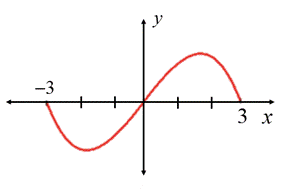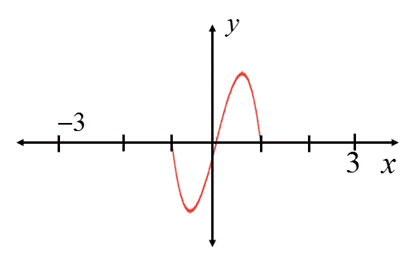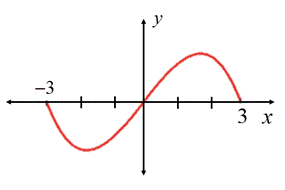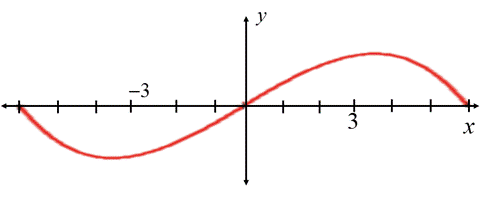# To graph the function.### Precalculus: Mathematics for Calcu...

6th Edition
Stewart + 5 others
Publisher: Cengage Learning
ISBN: 9780840068071### Precalculus: Mathematics for Calcu...

6th Edition
Stewart + 5 others
Publisher: Cengage Learning
ISBN: 9780840068071

#### Solutions

Chapter 2.5, Problem 66E

a.

To determine

## To graph the function.

Expert Solution

### Explanation of Solution

Given information:

The graph of h is given as:Concept used:

The horizontal shrinking and stretching of graph are defined by,

To graph, y=f(cx) ;

If c>1 , shrink the graph of y=f(x) horizontally by a factor of 1c .

If 0<c<1 , stretch the graph of y=f(x) horizontally by a factor of 1c .

Graph:

To graph y=h(3x) , use horizontal shrinking and stretching of graph,

Since, c=3>1 , so, the graph of y=h(3x) is obtained by shrinking the graph of f=h(x) horizontally by a factor of 13 .

Hence,

The graph of y=h(3x) is obtained as:Interpretation:

From, the above graph, the graph of y=h(3x) is obtained by shrinking the graph of f=h(x) horizontally by a factor of 13 .

b.

To determine

Expert Solution

### Explanation of Solution

Given information:

The graph of h is given as:Concept used:

The horizontal shrinking and stretching of graph are defined by,

To graph, y=f(cx) ;

If c>1 , shrink the graph of y=f(x) horizontally by a factor of 1c .

If 0<c<1 , stretch the graph of y=f(x) horizontally by a factor of 1c .

Graph:

To graph y=h(13x) , use horizontal shrinking and stretching of graph,

Since, 0<c=13<1 , so, the graph of y=h(13x) is obtained by stretching the graph of f=h(x) horizontally by a factor of 13 .

Hence,

The graph of y=h(13x) is obtained as:Interpretation:

From, the above graph, the graph of y=h(3x) is obtained by streching the graph of f=h(x) horizontally by a factor of 13 .

### Have a homework question?

Subscribe to bartleby learn! Ask subject matter experts 30 homework questions each month. Plus, you’ll have access to millions of step-by-step textbook answers!